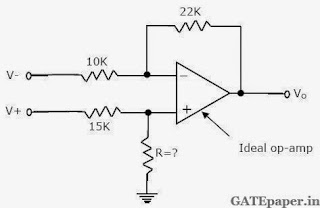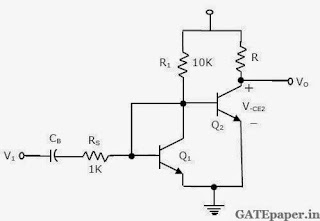### GATE 1995 ECE Analog Circuits (Analog Electronics) - Video Solutions

1. A change in the value of the emitter resistance, RE, in a differential amplifier
a. affects the difference mode gain Ad
b. affects the common mode gain Ac
c. affects both Ad and Ac
d. does not affect both Ad and Ac.

2. To obtain very high input and output impedance in a feedback amplifier, the topology mostly used is
a. Voltage Series
b. Current Series
c. Voltage Shunt
d. Current Shunt

3. In the given circuit, if the voltage V+ and V_ are to amplified by the same factor, the value of R should be _________4. An NPN transistor under forward active mode of operation is biased at Ic = 1 mA, and has a total emitter base capacitance CK of 12 pF, and the base transit time τF of 260 psec. Under this condition, the depletion capacitance of the emitter base junction is _____________

5. An RC coupled amplifier is assumed to have a single pole low frequency transfer function. The maximum lower cutoff frequency allowed for the amplifier to pass 50 Hz square wave with no more than 10% tilt is ______________

6. An OP-AMP is used as a zero crossing detector. If the maximum output available from the OP-AMP is ±12 volts peak to peak, and the slew rate of the OP-AMP is 12 V/µsec, then the maximum frequency of the input signal that can be applied without causing a reduction in the peak to peak output is _____________

7. A power amplifier delivers 50W output at 50% efficiency. The ambient temperature is 25oC. If the maximum allowable junction temperature is 150oC, then the maximum thermal resistance øjc that can be tolerated is __________

8. An amplifier has an open loop gain of 100, and its lower and upper cutoff frequency of 100 Hz and 100 kHz respectively. A feedback network with a feedback factor of 0.99 is connected to the amplifier. The new lower and upper cutoff frequencies are at __________ and ________

9. Match the following:
a) CC amplifier 1) provides voltage gain but no current gain
b) CE amplifier 2) provides current gain but no voltage gain
c) CB amplifier 3) provides neither voltage nor power gain
4) provides neither current nor power gain
5) provides both voltage and current gain

10. Sketch the output as a function of the input voltage (for negative values) for circuit shown below. Assume ideal operational amplifier and diode forward voltage drop as zero.11. The waveform input to the sweep generator circuit shown in figure, is a square wave of period 2 msec and amplitude varying between 0 and 12 volts.a. Draw the waveform Vo(t), in relation to the input
b. Specify Vo(t) determine the voltage levels and the time constants involved.

12. In the JFET circuit shown, assume that R1//R2 = 1 MΩ and the total stray capacitance at the output to be 20 pF.Determine the upper cutoff frequency of the amplifier.

13. Show that the circuit shown in figure is double integrator. In other words, prove that the transfer gain is given by Vo(s)/Vs(s) = 1/(SCR)2, assume ideal operational amplifier.14. In the amplifier circuit shown, determine the value of R such that Q2 is biased at VCE2 = 7.5 volts. Assume Q1 and Q2 to be identical with VBE = 0.7 volts.Also determine the small signal input impedance of Q1 and Q2, if both of them have β = 200. Use VT = 26 mV.